## Thursday, April 22, 2010

### Hadoop user group AKA Mahout Users Anonymous

At the Hadoop User Group meeting last evening it was quite the Mahout love fest.  First the Yahoo team described their spam fighting efforts which apparently are partially anchored by frequent item set mining using Mahout (thanks to Robin Anil's hard work as a GSoC student).  Then later Ken Krugler demonstrated some of what you can do with web-mining and Mahout.

These are just two of a growing number of real-world uses of Mahout which is really beginning to grow up.  Related to that growing up, the Apache board just decided to make Mahout a top level project.  That will take a little while to make real, but the first steps are already happening.

You can find out more about how Mahout is being used by perusing the Mahout wiki.

Slide decks available here for the HUG presentations.

## Tuesday, April 20, 2010

### Interview on Mahout available

I just had an interchange with InfoQ (as did Grant).  The results are to be found in the article on Mahout status that resulted.

## Saturday, April 10, 2010

### Sampling a Dirichlet Distribution (revised)

Last year, I casually wrote a post about how to sample from a prior for Dirichlet distributions.  There were recently a few comments on this blog and it now occurs to me that there is a massive simplification that is possible for the problem.

What I suggested back then was to sample the parameters of a Dirichlet distribution by sampling from a Dirichlet distribution and then multiplying that by a magnitude sampled from an exponential distribution.  As it turns out, this is a special case of a much nicer general method.

The new method is to sample each parameter independently from a gamma distribution
$\gamma_i \sim \mathrm{Gamma}(\alpha_i, \beta_i)$
This can be related to my previous method where we had the parameters expressed as a magnitude $\alpha$ multiplied by a vector $\vec m$ whose components sum to 1. Expressed in terms of the new method
$\alpha = \sum_i \gamma_i$
and
$m_i = \gamma_i / \alpha$
Moreover, if all of the $\beta_i$ are equal, then $\alpha$ is gamma distributed with shape parameter $\sum_i \alpha_i$. If this sum is 1, then we have an exponential distribution for $\alpha$ as in the original method.

I am pretty sure that this formulation also makes MCMC sampling from the posterior distribution easier as well because the products inside the expression for the joint probability will get glued together in a propitious fashion.

## Tuesday, April 6, 2010

### Sampling Dirichlet Distributions (chapter 2-b)

In the previous post, I talked about how sampling in soft-max space can make Metropolis algorithms work better for Dirichlet distributions. Here are some pictures that show why. These pictures are of three parameter Dirichlet distributions with the simplex projected into two dimensions. Dirichlet distributions with different parameters can look like this:

or this

or this

These were all generated using the standard algorithm for sampling from a Dirichlet distribution.

In R, that algorithm is this

rdirichlet = function(n, alpha) {
k = length(alpha)
r = matrix(0, nrow=n, ncol=k)
for (i in 1:k) {
r[,i] = rgamma(n, alpha[i], 1)
}
r = matrix(mapply(function(r, s) {return (r/s)}, r, rowSums(r)), ncol=k)
return (r)
}

That is, first sample $(y_1 \ldots y_n)$  using a gamma distribution
$y_i \sim \mathrm {Gamma} (\alpha_i, 1)$
Then normalize to get the desired sample
$\pi_i = {y_i \over \sum_j y_j}$
In contrast, here are 30,000 samples computed using a simple Metropolis algorithm to draw samples from a Dirichlet distribution with $\alpha = (1,1,1)$ .  This should give a completely uniform impression but there is noticeable thinning in the corners where the acceptance rate of the sampling process drops.
The thinning is only apparent.  In fact, what happens is that the samples in the corners are repeated, but that doesn't show up in this visual presentation.  Thus the algorithm is correct, but it exhibits very different mixing behavior depending on where you look.

A particularly unfortunate aspect of this problem is that decreasing the size of the step distribution in order to increase the acceptance rate in the corners makes the sampler take much longer to traverse the region of interest and thus only partially helps the problem.  Much of the problem is that when we have reached a point near the edge of the simplex or especially in a corner, many candidate steps will be off the simplex entirely and will thus be rejected.  When many parameters are small, this is particularly a problem because steps that are not rejected due to being off the simplex are likely to be rejected because the probability density drops dramatically as we leave the edge of the simplex.  Both of these problems become much, much worse in higher dimensions.

A much better alternative is to generate candidate points in soft-max space using a Gaussian step distribution.  This gives us a symmetric candidate distribution that takes small steps near the edges and corners of the simplex and larger steps in the middle of the range.  The sensitivity to the average step size (in soft-max space) is noticeably decreased and the chance of stepping out of the simplex is eliminated entirely.

This small cleverness leads to the solution of the question posed in a comment some time ago by Andrei about how to sample from the posterior distribution where we observe counts sampled from a multinomial
$\vec k \sim \mathrm {Multi} (\vec x)$
where that multinomial has a prior distribution defined by
\begin{aligned} \vec x &= \alpha \vec m \\ \alpha &\sim \mathrm{Exp} (\beta_0) \\ \vec m &\sim \mathrm{Dir} (\beta_1 \ldots \beta_n) \end{aligned}
More about that in my next post.

### Sampling Dirichlet Distributions (chapter 2-a)

In a previous post on sampling from Dirichlet distributions that related to a comment by Andrew Gelman on the same topic I talked a bit about how to sample the parameters of a Dirichlet (as opposed to sampling from a Dirichlet distribution with known parameters).

In responding to some questions and comments on my post, I went back and reread Andrew's thoughts and think that they should be amplified a bit.

Basically, what Andrew suggested is that when sampling from a Dirichlet, it is much easier to not sample from Dirichlet at all, but rather to sample from some unbounded distribution and then reduce that sample back to the unit simplex. The transformation that Andrew suggested is the same as the so-called soft-max basis that David Mackay also advocates for several purposes. This method is not well know, however, so it deserves a few more pixels.

The idea is that to sample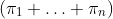from some distribution you instead sample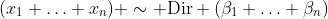and then reduce to the sample you want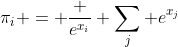Clearly this gives you values on the unit simplex. What is not clear is that this distribution is anything that you want. In fact, if your original sample is from a normal distribution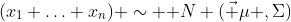then you can come pretty close to whatever desired Dirichlet distribution you like. More importantly in practice, this idea of normally sampling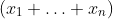and then transforming gives a very nice prior serves as well as a real Dirichlet distribution in many applications.

Where this comes in wonderfully handy is in MCMC sampling where you don't really want to sample from the prior, but instead want to sample from the posterior. It isn't all that hard to use the Dirichlet distribution directly in these cases, but the results are likely to surprise you. For instance, if you take the trivial case of picking a uniformly distributed point on the simplex and then jumping around using Metropolis updates based on a Dirichlet distribution, you will definitely have the right distribution for your samples after just a bit of sampling. But if you plot those points, they won't look at all right if you are sampling from a distribution that has any significant density next to the boundaries of the simplex. What is happening is that the high probability of points near the boundaries is accounted for in the Metropolis algorithm by having a high probability of rejecting any jump if you are near the boundary. Thus, the samples near the boundary are actually repeated many times leading to the visual impression of low density where there should be high density.

On the other hand, if you were to do the Metropolis jumps in the soft-max basis, the problem is entirely avoided because there are no boundaries in soft-max space. You can even use the soft-max basis for the sole purpose of generating candidate points and do all probability calculations in terms on the simplex.

In my next post, I will provide a concrete example and even include some pictures.

## Sunday, April 4, 2010

### Big Data and Big Problems

In talks recently, I have mentioned an ACM paper that showed just how bad random access to various storage devices can be.  About half the time I mention this paper, somebody asks for the reference which I never know off the top of my head.  So here it is

The Pathologies of Big Data

Of course, the big deal is not that disks are faster when read sequentially.  We all know that and most people I talk to know that the problem is worse than you would think.  The news is that random access to memory is much, much worse than most people would imagine.  In the slightly contrived example in this paper, for instance, sequential reads from a hard disk deliver data faster than randomized reads from RAM.  That is truly horrifying.

Even though you can pick holes in the example, it shows by mere existence that the problem of random access is much worse than almost anybody has actually internalized.